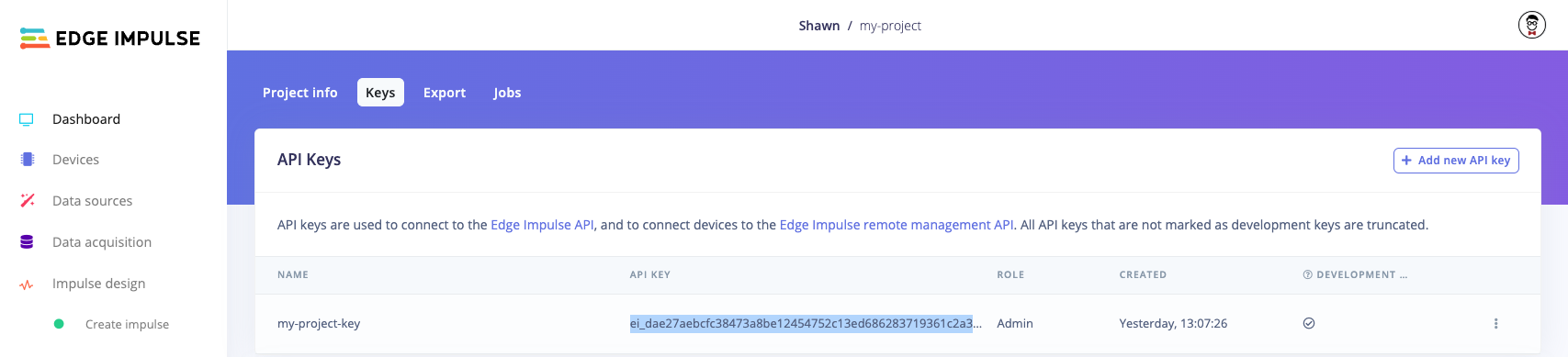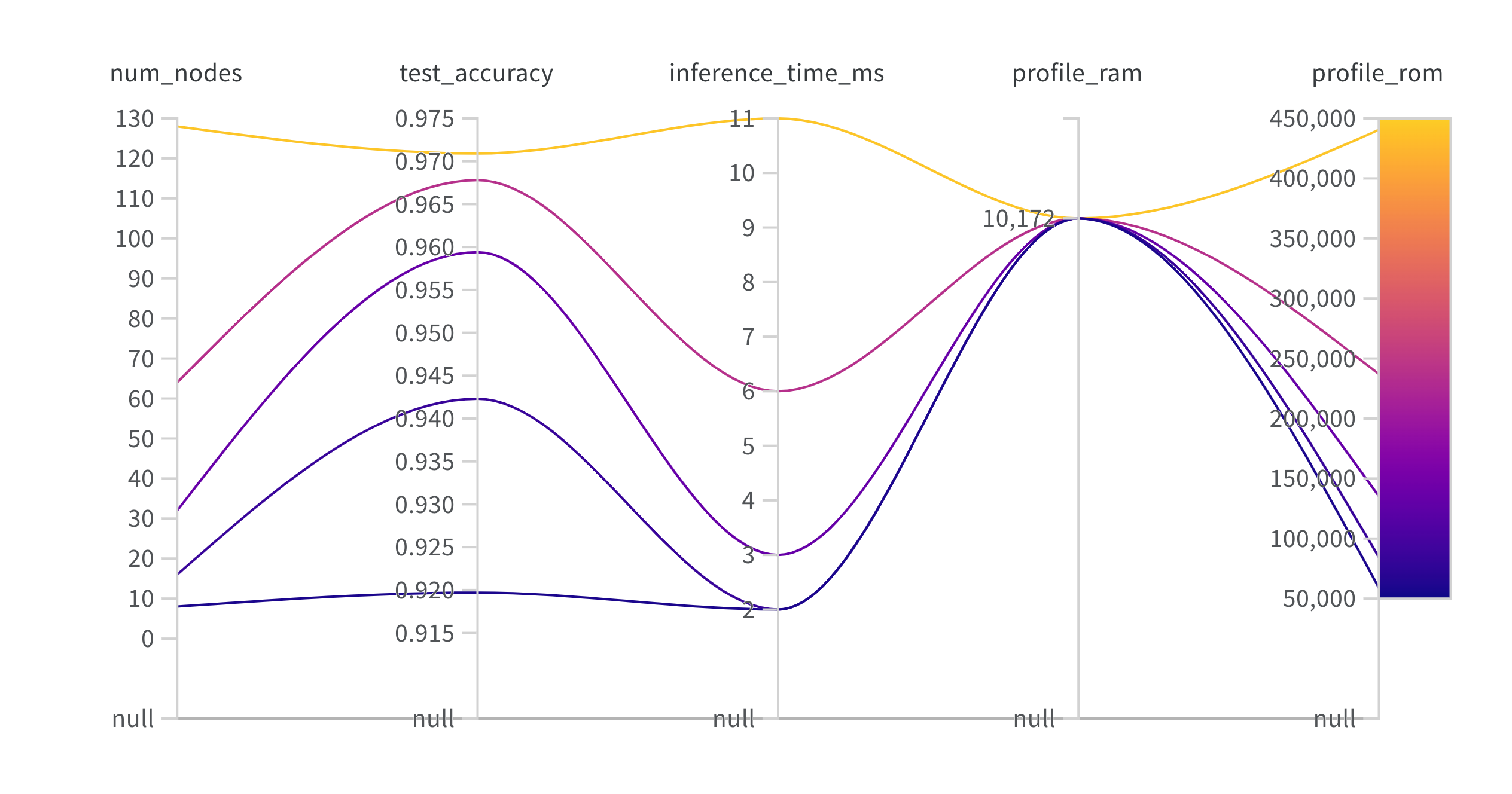# Using the Edge Impulse Python SDK with Weights & Biases

Weights & Biases is an online framework for helping manage machine learning training, data versioning, and experiments. When running experiments for edge-focused ML projects, it can be helpful to see the required memory (RAM and ROM) along with estimated inference times of your model for your target hardware. By viewing these metrics, you can quickly gauge if your model will fit onto your target device!
Follow the code below to see how to train a simple machine learning model with different hyperparameters and log those values to the Weights & Biases dashboard.
# If you have not done so already, install the following dependencies
!python -m pip install tensorflow==2.12.0 wandb edgeimpulse
from tensorflow import keras
import wandb
import edgeimpulse as ei
You will need to obtain an API key from an Edge Impulse project. Log into edgeimpulse.com and create a new project. Open the project, navigate to Dashboard and click on the Keys tab to view your API keys. Double-click on the API key to highlight it, right-click, and select Copy.Copy API key from Edge Impulse project
Note that you do not actually need to use the project in the Edge Impulse Studio. We just need the API Key.
Paste that API key string in the `ei.API_KEY` value in the following cell:
# Settings %%%RESET API_KEY, project_name
ei.API_KEY = "ei_dae2..." # Change this to your Edge Impulse API key
labels = ["0", "1", "2", "3", "4", "5", "6", "7", "8", "9"]
num_classes = len(labels)
num_epochs = 5
profile_device = 'cortex-m4f-80mhz' # Run ei.model.list_profile_devices() to see available devices
deploy_filename = "my_model_cpp.zip"
# Define experiment hyperparameters - sweep across number of nodes
project_name = "nodes-sweep"
num_nodes_sweep = [8, 16, 32, 64, 128]
To use Weights and Biases, you will need to create an account on wandb.ai and call the `wandb.login()` function. This will prompt you to log in to your account. Your credentials should be stored, which allows you to use the `wandb` package in your Python library.

## Gather a dataset

We want to create a classifier that can uniquely identify handwritten digits. To start, we will use TensorFlow and Keras to train a very simple convolutional neural network (CNN) on the classic MNIST dataset, which consists of handwritten digits from 0 to 9.
(x_train, y_train), (x_test, y_test) = keras.datasets.mnist.load_data()
x_train = keras.utils.normalize(x_train, axis=1)
x_test = keras.utils.normalize(x_test, axis=1)
y_train = keras.utils.to_categorical(y_train, num_classes)
y_test = keras.utils.to_categorical(y_test, num_classes)
input_shape = x_train.shape

## Create an experiment

We want to vary the hyperparameters in our model and see how it affects the accuracy and predicted RAM, ROM, and inference time on our target platform. To do that, we construct a function that builds a simple model using Keras, trains the model, and computes the accuracy and loss from our holdout test set. We then use the Edge Impulse Python SDK to generate a profile of our model for our target hardware. We log the hyperparameter (number of nodes in the hidden layer), test loss, test accuracy, estimated RAM, estimated ROM, and estimated inference time (ms) to our Weights and Biases console.
# Define experiment - Train and test model, log metrics
def do_experiment(num_nodes):
# Create W&B project
run = wandb.init(project=project_name,
name=f"{num_nodes}-nodes")
# Build the model (vary number of nodes in the hidden layer)
model = keras.Sequential([
keras.layers.Flatten(),
keras.layers.Dense(num_nodes, activation='relu', input_shape=input_shape),
keras.layers.Dense(num_classes, activation='softmax')
])
# Compile the model
loss='categorical_crossentropy',
metrics=['accuracy'])
# Train the model
model.fit(x_train,
y_train,
epochs=num_epochs)
# Evaluate model
test_loss, test_accuracy = model.evaluate(x_test, y_test)
# Profile model on target device
try:
profile = ei.model.profile(model=model,
device=profile_device)
except Exception as e:
print(f"Could not profile: {e}")
# Log metrics
if profile.success:
print("Profiling successful. Logging.")
wandb.log({
'num_nodes': num_nodes,
'test_loss': test_loss,
'test_accuracy': test_accuracy,
'profile_ram': profile.model.profile_info.float32.memory.tflite.ram,
'profile_rom': profile.model.profile_info.float32.memory.tflite.rom,
'inference_time_ms': profile.model.profile_info.float32.time_per_inference_ms
})
else:
print(f"Profiling unsuccessful. Error: {job_resp.error}")
# Close run
wandb.finish()

## Run the experiment

Now, it's time to run the experiment and log the results in Weights and Biases. Simply call our function and provide a new hyperparameter value for the number of nodes.
# Perform the experiments - check your dashboard in WandB!
for num_nodes in num_nodes_sweep:
do_experiment(num_nodes)
Head to wandb.ai and log in (if you have not already done so). Under My projects on the left, click on the nodes-sweep project. You can visualize the results of your experiments with the various charts that Weights & Biases offers. For example, here is a parallel coordinates plot that allows you to quickly visualize the different hyperparameters and metrics (including our new edge profile metrics).Weights and Biases parallel coordinates plot
If you would like to deploy your model to your target hardware, the Python SDK can help you with that, too. See our documentation here.

Once you are happy with the performance of your model, you can then deploy it to your target hardware. We will assume that 32 nodes in our hidden layer provided the best trade-off of RAM, flash, inference time, and accuracy for our needs. To start, we will retrain the model:
# Build the model
model = keras.Sequential([
keras.layers.Flatten(),
keras.layers.Dense(32, activation='relu', input_shape=input_shape),
keras.layers.Dense(num_classes, activation='softmax')
])
# Compile the model
loss='categorical_crossentropy',
metrics=['accuracy'])
# Train the model
model.fit(x_train,
y_train,
epochs=5)
Next, we should evaluate the model on our holdout test set.
# Evaluate model on test set
score = model.evaluate(x_test, y_test, verbose=0)
print(f"Test loss: {score}")
print(f"Test accuracy: {score}")
From there, we can see the available hardware targets for deployment:
# List the available profile target devices
ei.model.list_deployment_targets()
You should see a list printed such as:
['zip',
'arduino',
'tinkergen',
'cubemx',
'wasm',
...
'runner-linux-aarch64-tda4vm']
The most generic target is the .zip file that holds a C++ library containing our trained model and inference runtime. To pass our labels to the C++ library, we create a Classification object, which contains our label strings.
Note that instead of writing the raw bytes to a file, you can also specify an `output_directory` argument in the .deploy() function. Your deployment file(s) will be downloaded to that directory.
# Set model information, such as your list of labels
model_output_type = ei.model.output_type.Classification(labels=labels)
# Create C++ library with trained model
deploy_bytes = None
try:
deploy_bytes = ei.model.deploy(model=model,
model_output_type=model_output_type,
deploy_target='zip')
except Exception as e:
print(f"Could not deploy: {e}")